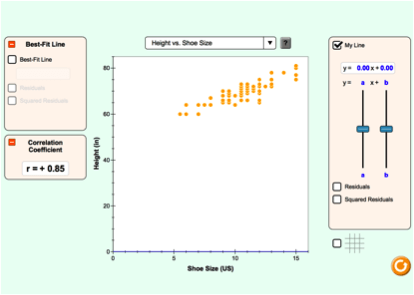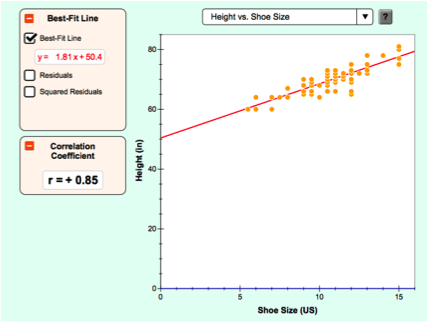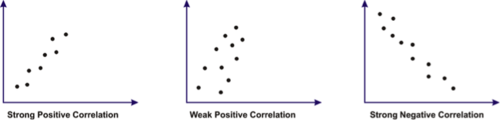#### Is the average date for hatching getting earlier?

Above is a graph showing the average date that Pacific Black Brant geese hatched in the Yukon Kuskokwim Delta since 1983. Is there a trend? Is this date getting earlier? Does this data suggest that geese are arriving earlier on the delta each year? How do scientist know when the data can be used as evidence with confidence?

### Vocabulary

• correlation
• best fit line
• scatter plot

### Learn Objective

• Use a simulation to create a scatter plot and draw a line of best fit for the data.
• Make predictions using a line of best fit to describe the correlation of variables in collected data.

### Scatter Plots

Scientist often use a scatterplot to analyze the relationship between two variables that they test. Here you see a scatterplot that graphs data points on shoe size of the population sampled versus their height.### Best Fit Lines

A best fit line is drawn in the middle of all the data points. The slope of this line shows the relationship of the data. If a clear relationship exists, it is called a correlation.[Figure3]

When correlation exists on a scatter plot, a line of best fit can be drawn on the graph. Draw a best fit line through the following data points. The higher the slope, the stronger the correlation. The correlation can be calculated mathematically by determining the slope of the best fit line.Data with a weak correlation will have a flatter slope that is a lower or closer to 0.

To understand this concept better, we will look at some scatter plots and slopes in the PHET model. The correlation coefficients have been calculated for these data sets.Download The Handout , ” Interpreting the Relationships Between Two Variables”. Read the instructions and follow the directions to learn how to use this technique to analyze data.

Data with a weak correlation will have a flatter slope that is a lower or closer to 0.
Once you have studied how a best fit line helps us look for trends and relationships in science, look at the spring hatch data with the trend line. Is there a correlation? Is it a strong correlation?